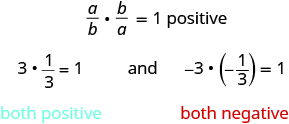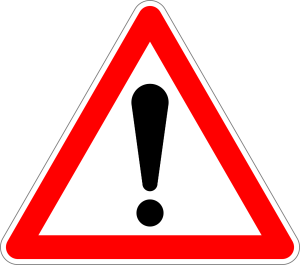## Finding the Reciprocal of a Number

### Learning Outcomes

• Find the reciprocal of a fraction

The fractions $\Large\frac{2}{3}$ and $\Large\frac{3}{2}$ are related to each other in a special way. So are $\Large-\frac{10}{7}$ and $\Large-\frac{7}{10}$. Do you see how? Besides looking like upside-down versions of one another, if we were to multiply these pairs of fractions, the product would be $1$.

$\Large\frac{2}{3}\cdot \frac{3}{2}\normalsize=1\text{ and }-\Large\frac{10}{7}\left(-\frac{7}{10}\right)\normalsize=1$

Such pairs of numbers are called reciprocals.

### Reciprocal

The reciprocal of the fraction $\Large\frac{a}{b}$ is $\Large\frac{b}{a}$, where $a\ne 0$ and $b\ne 0$,
A number and its reciprocal have a product of $1$.

$\Large\frac{a}{b}\cdot \frac{b}{a}\normalsize=1$

Here are some examples of reciprocals:

Original number Reciprocal Product
$\dfrac{3}{4}$ $\dfrac{4}{3}$ $\dfrac{3}{4}\cdot\dfrac{4}{3}=\dfrac{3\cdot 4}{4\cdot 3}=\dfrac{12}{12}=1$
$\dfrac{1}{2}$ $\dfrac{2}{1}$ $\dfrac{1}{2}\cdot\dfrac{2}{1}=\dfrac{1\cdot2}{2\cdot1}=\dfrac{2}{2}=1$
$3=\dfrac{3}{1}$ $\dfrac{1}{3}$ $\dfrac{3}{1}\cdot\dfrac{1}{3}=\dfrac{3\cdot 1}{1\cdot 3}=\dfrac{3}{3}=1$
$2\dfrac{1}{3}=\dfrac{7}{3}$ $\dfrac{3}{7}$ $\dfrac{7}{3}\cdot\dfrac{3}{7}=\dfrac{7\cdot3}{3\cdot7}=\dfrac{21}{21}=\normalsize 1$

To find the reciprocal of a fraction, we invert the fraction. This means that we place the numerator in the denominator and the denominator in the numerator.  You can think of it as switching the numerator and denominator: swap the $2$ with the $5$ in $\dfrac{2}{5}$ to get the reciprocal $\dfrac{5}{2}$.

Make sure that if it’s a negative fraction, the reciprocal is also negative. This is because the product of two negative numbers will give you the positive one that you are looking for.  To get a positive result when multiplying two numbers, the numbers must have the same sign. So reciprocals must have the same sign.To find the reciprocal, keep the same sign and invert the fraction.

### Example

Find the reciprocal of each number. Then check that the product of each number and its reciprocal is $1$.

1. $\Large\frac{4}{9}$
2. $\Large-\frac{1}{6}$
3. $\Large-\frac{14}{5}$
4. $7$

Solution:
To find the reciprocals, we keep the sign and invert the fractions.

 1. Find the reciprocal of $\Large\frac{4}{9}$ The reciprocal of $\Large\frac{4}{9}$ is $\Large\frac{9}{4}$ Check: Multiply the number and its reciprocal. $\Large\frac{4}{9}\cdot \frac{9}{4}$ Multiply numerators and denominators. $\Large\frac{36}{36}$ Simplify. $1\quad\checkmark$
 2. Find the reciprocal of $\Large-\frac{1}{6}$ $\Large-\frac{6}{1}$ Simplify. $-6$ Check: $\Large-\frac{1}{6}\normalsize\cdot \left(-6\right)$ $1\quad\checkmark$
 3. Find the reciprocal of $\Large-\frac{14}{5}$ $\Large-\frac{5}{14}$ Check: $\Large-\frac{14}{5}\cdot \left(-\frac{5}{14}\right)$ $\Large\frac{70}{70}$ $1\quad\checkmark$
 4. Find the reciprocal of $7$ Write $7$ as a fraction. $\Large\frac{7}{1}$ Write the reciprocal of $\Large\frac{7}{1}$ $\Large\frac{1}{7}$ Check: $7\cdot\Large\left(\frac{1}{7}\right)$ $1\quad\checkmark$

### Try It

In the following video we will show more examples of how to find the reciprocal of integers, fractions and mixed numbers.Caution! Division by zero is undefined and so is the reciprocal of any fraction that has a zero in the numerator. For any real number a, $\dfrac{a}{0}$ is undefined. Additionally, the reciprocal of $\dfrac{0}{a}$ will always be undefined.

## Division by Zero

You know what it means to divide by $2$ or divide by $10$, but what does it mean to divide a quantity by $0$? Is this even possible? On the flip side, can you divide $0$ by a number? Consider the fraction

$\dfrac{0}{8}$

We can read it as, “zero divided by eight.” Since multiplication is the inverse of division, we could rewrite this as a multiplication problem. What number times $8$ equals $0$?

$\text{?}\cdot{8}=0$

We can infer that the unknown must be $0$ since that is the only number that will give a result of $0$ when it is multiplied by $8$.

Now let’s consider the reciprocal of $\dfrac{0}{8}$ which would be $\dfrac{8}{0}$. If we rewrite this as a multiplication problem, we will have “what times $0$ equals $8$?”

$\text{?}\cdot{0}=8$

This doesn’t make any sense. There are no numbers that you can multiply by zero to get a result of 8. In fact, any number divided by $0$ is impossible, or better defined, all division by zero is undefined.

## Contribute!

Did you have an idea for improving this content? We’d love your input.